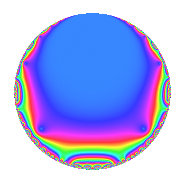Properties

 Label 8001.2.a.fLevel 8001 Weight 2 Character orbit 8001.a Self dual Yes Analytic conductor 63.888 Analytic rank 1 Dimension 1 CM No Inner twists 1

Related objects

Newspace parameters

 Level: $$N$$ = $$8001 = 3^{2} \cdot 7 \cdot 127$$ Weight: $$k$$ = $$2$$ Character orbit: $$[\chi]$$ = 8001.a (trivial)

Newform invariants

 Self dual: Yes Analytic conductor: $$63.8883066572$$ Analytic rank: $$1$$ Dimension: $$1$$ Coefficient field: $$\mathbb{Q}$$ Coefficient ring: $$\mathbb{Z}$$ Coefficient ring index: $$1$$ Fricke sign: $$1$$ Sato-Tate group: $\mathrm{SU}(2)$

$q$-expansion

 $$f(q)$$ $$=$$ $$q$$ $$\mathstrut +\mathstrut 2q^{2}$$ $$\mathstrut +\mathstrut 2q^{4}$$ $$\mathstrut -\mathstrut q^{7}$$ $$\mathstrut +\mathstrut O(q^{10})$$ $$q$$ $$\mathstrut +\mathstrut 2q^{2}$$ $$\mathstrut +\mathstrut 2q^{4}$$ $$\mathstrut -\mathstrut q^{7}$$ $$\mathstrut +\mathstrut 2q^{13}$$ $$\mathstrut -\mathstrut 2q^{14}$$ $$\mathstrut -\mathstrut 4q^{16}$$ $$\mathstrut -\mathstrut q^{17}$$ $$\mathstrut -\mathstrut 2q^{19}$$ $$\mathstrut -\mathstrut 5q^{25}$$ $$\mathstrut +\mathstrut 4q^{26}$$ $$\mathstrut -\mathstrut 2q^{28}$$ $$\mathstrut +\mathstrut 9q^{29}$$ $$\mathstrut -\mathstrut 8q^{32}$$ $$\mathstrut -\mathstrut 2q^{34}$$ $$\mathstrut -\mathstrut q^{37}$$ $$\mathstrut -\mathstrut 4q^{38}$$ $$\mathstrut +\mathstrut 3q^{41}$$ $$\mathstrut -\mathstrut 6q^{43}$$ $$\mathstrut -\mathstrut 8q^{47}$$ $$\mathstrut +\mathstrut q^{49}$$ $$\mathstrut -\mathstrut 10q^{50}$$ $$\mathstrut +\mathstrut 4q^{52}$$ $$\mathstrut -\mathstrut 11q^{53}$$ $$\mathstrut +\mathstrut 18q^{58}$$ $$\mathstrut +\mathstrut 12q^{59}$$ $$\mathstrut -\mathstrut 10q^{61}$$ $$\mathstrut -\mathstrut 8q^{64}$$ $$\mathstrut +\mathstrut 4q^{67}$$ $$\mathstrut -\mathstrut 2q^{68}$$ $$\mathstrut -\mathstrut 8q^{71}$$ $$\mathstrut -\mathstrut 4q^{73}$$ $$\mathstrut -\mathstrut 2q^{74}$$ $$\mathstrut -\mathstrut 4q^{76}$$ $$\mathstrut -\mathstrut 13q^{79}$$ $$\mathstrut +\mathstrut 6q^{82}$$ $$\mathstrut +\mathstrut 6q^{83}$$ $$\mathstrut -\mathstrut 12q^{86}$$ $$\mathstrut -\mathstrut 12q^{89}$$ $$\mathstrut -\mathstrut 2q^{91}$$ $$\mathstrut -\mathstrut 16q^{94}$$ $$\mathstrut -\mathstrut 7q^{97}$$ $$\mathstrut +\mathstrut 2q^{98}$$ $$\mathstrut +\mathstrut O(q^{100})$$

Embeddings

For each embedding $$\iota_m$$ of the coefficient field, the values $$\iota_m(a_n)$$ are shown below.

For more information on an embedded modular form you can click on its label.

Label $$\iota_m(\nu)$$ $$a_{2}$$ $$a_{3}$$ $$a_{4}$$ $$a_{5}$$ $$a_{6}$$ $$a_{7}$$ $$a_{8}$$ $$a_{9}$$ $$a_{10}$$
1.1
 0
2.00000 0 2.00000 0 0 −1.00000 0 0 0
 $$n$$: e.g. 2-40 or 990-1000 Significant digits: Format: Complex embeddings Normalized embeddings Satake parameters Satake angles

Inner twists

This newform does not admit any (nontrivial) inner twists.

Atkin-Lehner signs

$$p$$ Sign
$$3$$ $$-1$$
$$7$$ $$1$$
$$127$$ $$-1$$

Hecke kernels

This newform can be constructed as the intersection of the kernels of the following linear operators acting on $$S_{2}^{\mathrm{new}}(\Gamma_0(8001))$$:

 $$T_{2}$$ $$\mathstrut -\mathstrut 2$$ $$T_{5}$$